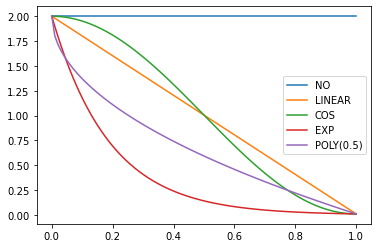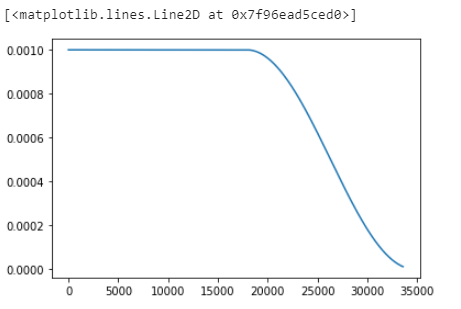# Passing Iteration number to LambdaLR

How i can pass iteration to Lambda LR instead of Epoch

I want to varry my LR using this function after running it with flat LR for certain number of iterations

`def sched_cos(start, end, pos): return start + (1 + math.cos(math.pi*(1-pos))) * (end-start) / 2`

Where pos=iteration number

My objective is to train with Flat LR for certaion portion of training cycle say 30 percent then there after decay the LR as per cosine function … Some thing like that as shown in image. Green Line.I think i found solution

``````model = nn.Linear(10, 2)
optimizer = optim.SGD(model.parameters(), lr=1e-3)
steps = 10

scheduler=CosineAnnealingLR(optimizer, T_max=15*2240//2, eta_min=0, last_epoch=-1,
verbose=False)
lrs=[]
for epoch in range(15):
for idx in range(2240):
if epoch>15//2:

scheduler.step()
#print(scheduler.get_last_lr()[-1])
lrs.append(scheduler.get_last_lr()[-1])
``````